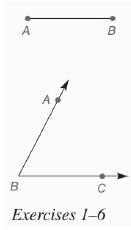Chapter 7.CR, Problem 5CRElementary Geometry For College St...

7th Edition
Alexander + 2 others
ISBN: 9781337614085

Solutions

Chapter
SectionElementary Geometry For College St...

7th Edition
Alexander + 2 others
ISBN: 9781337614085
Textbook Problem

In Review Exercises 1 to 6, use the figure shown.Construct a square with sides of length A B .To determine

To construct: A square with sides of length AB.

Explanation

Given:

The given figure is shown below:

Calculation:

First, extend the line AB to the right.

Now, draw an arc on each side B using any compass width. Label F and G.

Without changing the width, repeat from point F, creating point H...

Still sussing out bartleby?

Check out a sample textbook solution.

See a sample solution

The Solution to Your Study Problems

Bartleby provides explanations to thousands of textbook problems written by our experts, many with advanced degrees!

Get Started

Evaluate each expression: 20+(23)2723

Elementary Technical Mathematics

In Exercises 39-54, simplify the expression. (Assume that x, y, r, s, and t are positive.) 40. (49x2)1/2

Applied Calculus for the Managerial, Life, and Social Sciences: A Brief Approach

Prove each identity. sin()sec()cot()=1

Trigonometry (MindTap Course List)

π does not exist

Study Guide for Stewart's Multivariable Calculus, 8th